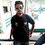# Does the distributed property of modular arithematic have a proof?

There is a property in modular arithematic and that is:

(a + b) mod N ≡ a mod N + b mod N

I wonder if there is a proof why this mod distributes inside brackets.

Any help would be really appreciated.

Pardon if this is a silly question.Note by Shiran Abbasi
7 months, 3 weeks ago

This discussion board is a place to discuss our Daily Challenges and the math and science related to those challenges. Explanations are more than just a solution — they should explain the steps and thinking strategies that you used to obtain the solution. Comments should further the discussion of math and science.

When posting on Brilliant:

• Use the emojis to react to an explanation, whether you're congratulating a job well done , or just really confused .
• Ask specific questions about the challenge or the steps in somebody's explanation. Well-posed questions can add a lot to the discussion, but posting "I don't understand!" doesn't help anyone.
• Try to contribute something new to the discussion, whether it is an extension, generalization or other idea related to the challenge.
• Stay on topic — we're all here to learn more about math and science, not to hear about your favorite get-rich-quick scheme or current world events.

MarkdownAppears as
*italics* or _italics_ italics
**bold** or __bold__ bold
- bulleted- list
• bulleted
• list
1. numbered2. list
1. numbered
2. list
Note: you must add a full line of space before and after lists for them to show up correctly
paragraph 1paragraph 2

paragraph 1

paragraph 2

[example link](https://brilliant.org)example link
> This is a quote
This is a quote
    # I indented these lines
# 4 spaces, and now they show
# up as a code block.

print "hello world"
# I indented these lines
# 4 spaces, and now they show
# up as a code block.

print "hello world"
MathAppears as
Remember to wrap math in $$ ... $$ or $ ... $ to ensure proper formatting.
2 \times 3 $2 \times 3$
2^{34} $2^{34}$
a_{i-1} $a_{i-1}$
\frac{2}{3} $\frac{2}{3}$
\sqrt{2} $\sqrt{2}$
\sum_{i=1}^3 $\sum_{i=1}^3$
\sin \theta $\sin \theta$
\boxed{123} $\boxed{123}$

## Comments

Sort by:

Top Newest

I don't think it will because

$(7 + 10) \mod 4 \equiv 7 \mod 4 + 10 \mod 4 \equiv 3 + 2 \equiv 5$

but $5$ is not $17 \mod 4 \equiv 1$, so I don't think it applies. Please correct me if I'm wrong.

- 7 months, 3 weeks ago

Log in to reply

You're wrong. $5\equiv 1 \pmod4$.

- 7 months, 3 weeks ago

Log in to reply

I was disproving the property that Shiran provided. By separating 17 into 7+10 and modding both of them.

- 7 months, 3 weeks ago

Log in to reply

The equation he provided is correct.

17 mod 4 == (7 mod 4) + (10 mod4)

Notice the equivalent symbol in the middle, it's not an equal sign.

- 7 months, 3 weeks ago

Log in to reply

Now I'm confused.

$17 \mod 4 \equiv 1$ because 17 = 4x4 + 1

So to find - $17 \mod 4 \equiv (7 \mod 4) + (10 \mod 4)$

we know $(7 \mod 4) \equiv 3 \text{ and } (10 \mod 4) \equiv 2$

Shouldn't $(7 \mod 4) + (10 \mod 4) \equiv 3 + 2 \equiv 5$???

If we calculate (7 mod 4) and (10 mod 4) separately, and then add them, we get 5 don't we???

- 7 months, 3 weeks ago

Log in to reply

the source is this: https://brilliant.org/wiki/modular-arithmetic/#modular-arithmetic-addition

- 7 months, 3 weeks ago

Log in to reply

≡ sign here means congruence.

So, referring to your first comment:

17 ≡ 5 ≡ 1 mod 4

- 7 months, 3 weeks ago

Log in to reply

i also asked this question on math.stackexchange but they closed my question. Unfortunately i can't find an answer to my question.

Can anyone help?

- 7 months, 3 weeks ago

Log in to reply

You're confusing the two notations $\pmod {x}$ and $\bmod x$.

If $A \bmod B = C$, then $A \pmod B \equiv C$ as well. Similarly, $A\pmod B \equiv C + Bn$, where $n$ is any integer.

For example, $17\bmod4 = 1$, and $17\pmod4 \equiv 1$ are true.

Similarly,
$17\pmod 4 \equiv 5$,
$17\pmod 4 \equiv 9$,
$17\pmod 4 \equiv -3$,
$17\pmod 4 \equiv -7$ are all true as well.

By definition, if $A \pmod B \equiv C$, then $A-C$ is divisible by $B$.

On the other hand, $A \bmod B = C$ implies that when $A$ is divided by $B$, the remainder is $C$.

Do you see the subtle difference here?

- 7 months, 3 weeks ago

Log in to reply

thanks for your answer. Now i got it.

- 7 months, 3 weeks ago

Log in to reply

Thanks @Pi Han Goh, I understood now!

- 7 months, 3 weeks ago

Log in to reply

×

Problem Loading...

Note Loading...

Set Loading...